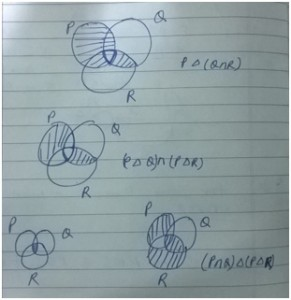GATE | GATE IT 2006 | Question 23

• Last Updated : 28 Jun, 2021

Let P, Q and R be sets let Δ denote the symmetric difference operator defined as PΔQ = (P U Q) – (P ∩ Q). Using Venn diagrams, determine which of the following is/are TRUE?

PΔ (Q ∩ R) = (P Δ Q) ∩ (P Δ R)
P ∩ (Q ∩ R) = (P ∩ Q) Δ (P Δ R)

(A) I only
(B) II only
(C) Neither I nor II
(D) Both I and II

Explanation: